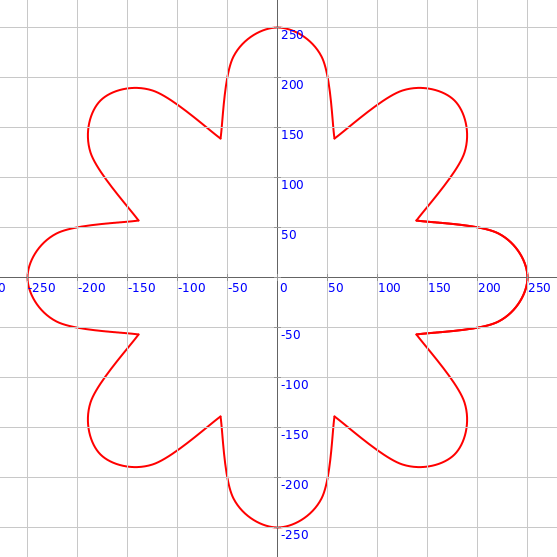## Mandala Building Blocks - 1

This activity has the following desired goals:

• Learning about the different pre-defined mandala building blocks (A, M).

### Building Block 1 - Lotus Petal

Type in the following code and run it:

``````def lotusPetal(radius: Double, radiusOuter: Double,
theta: Double, thetaExtent: Double) = Picture.fromVertexShape { s =>
import s._
val tDelta = thetaExtent / 2
beginShape()

curveVertexRt(mathx.lerp(radius, radius * rExtent, 0.6), theta - tDelta * 22 / 30)
curveVertexRt(mathx.lerp(radius, radius * rExtent, 0.7), theta - tDelta * 2 / 30)
curveVertexRt(mathx.lerp(radius, radius * rExtent, 0.7), theta + tDelta * 2 / 30)
curveVertexRt(mathx.lerp(radius, radius * rExtent, 0.6), theta + tDelta * 22 / 30)

endShape()
}

cleari()
showAxes()
showGrid()
val pic = lotusPetal(150, 200, 0, 60)
draw(pic)
``````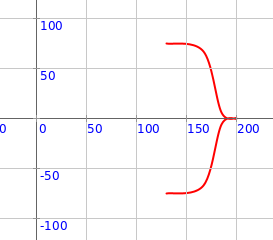Q1a. The code above makes one lotus petal. What is its radius? What is its outer radius? What is its direction (theta)? What is it’s spread (thetaExtent)?

#### Exploration

Play with the inputs to the `lotusPetal` function above to make different kinds of petals.

Type in the following code and run it:

``````def lotusPetal(radius: Double, radiusOuter: Double,
theta: Double, thetaExtent: Double) = Picture.fromVertexShape { s =>
import s._
val tDelta = thetaExtent / 2
beginShape()

curveVertexRt(mathx.lerp(radius, radius * rExtent, 0.6), theta - tDelta * 22 / 30)
curveVertexRt(mathx.lerp(radius, radius * rExtent, 0.7), theta - tDelta * 2 / 30)
curveVertexRt(mathx.lerp(radius, radius * rExtent, 0.7), theta + tDelta * 2 / 30)
curveVertexRt(mathx.lerp(radius, radius * rExtent, 0.6), theta + tDelta * 22 / 30)

endShape()
}

cleari()
showAxes()
showGrid()
val pics = ArrayBuffer.empty[Picture]
repeatFor(0 to 6) { n =>
val pic = lotusPetal(150, 200, n * 60, 60)
pics.append(pic)
}
draw(pics)
``````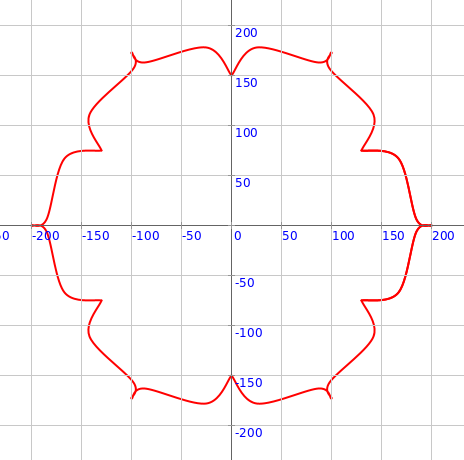Q1b. How does the above code make lotus petals in a circular pattern?

#### Exploration

Play with the above code to make different kinds of circular patterns

### Building Block 2 - Diya

Type in the following code and run it:

``````def diya(radius: Double, radiusOuter: Double,
theta: Double, thetaExtent: Double) = Picture.fromVertexShape { s =>
import s._
val tDelta = thetaExtent / 2
beginShape()
endShape()

beginShape()
endShape()
}

cleari()
showAxes()
showGrid()
val pic = diya(150, 250, 0, 50)
draw(pic)
``````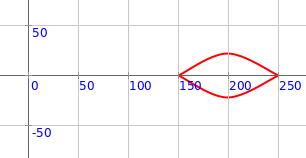Q2a. The code above makes one diya. What is its radius? What is its outer radius? What is its direction (theta)? What is it’s spread (thetaExtent)?

#### Exploration

Play with the inputs to the `diya` function above to make different kinds of diyas.

#### Exercise

Make the following circular pattern: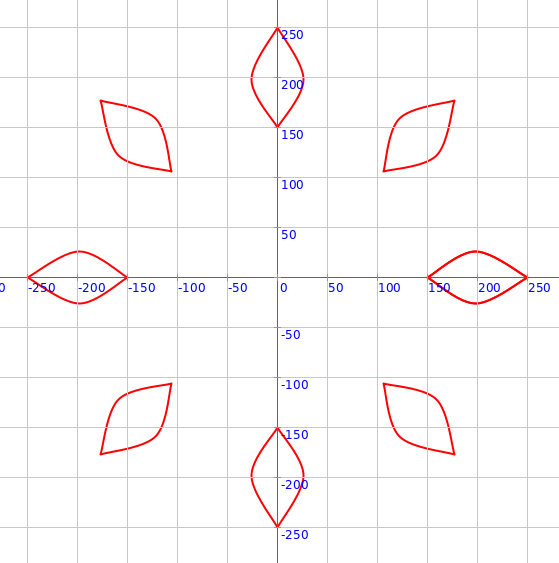### Building Block 3 - Pointed Petal

Type in the following code and run it:

``````def pointedPetal(radius: Double, radiusOuter: Double,
theta: Double, thetaExtent: Double) = Picture.fromVertexShape { s =>
val tDelta = thetaExtent / 2
import s._
beginShape()

endShape()
}

cleari()
showAxes()
showGrid()
val pic = pointedPetal(150, 250, 0, 60)
draw(pic)
``````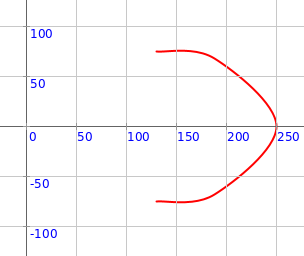Q3a. The code above makes one pointed petal. What is its radius? What is its outer radius? What is its direction (theta)? What is it’s spread (thetaExtent)?

#### Exploration

Play with the inputs to the `pointedPetal` function above to make different kinds of petals.

#### Exercise

Make the following circular pattern: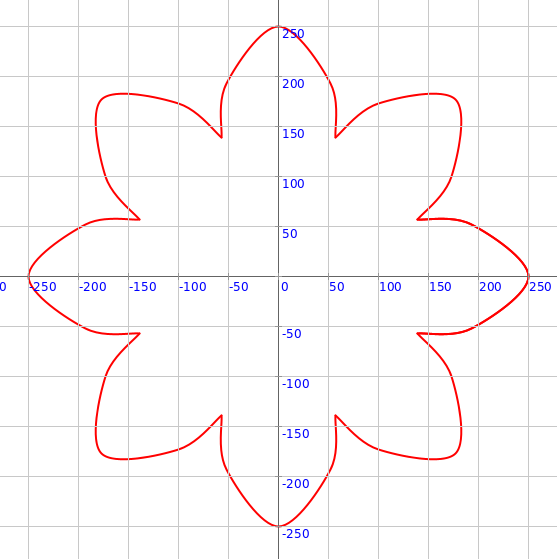### Building Block 4 - Rounded Petal

Type in the following code and run it:

``````def roundedPetal(radius: Double, radiusOuter: Double,
theta: Double, thetaExtent: Double) = Picture.fromVertexShape { s =>
val tDelta = thetaExtent / 2
import s._
implicit val s2 = s
beginShape()

endShape()
}

cleari()
showAxes()
showGrid()
val pic = roundedPetal(150, 250, 0, 40)
draw(pic)
``````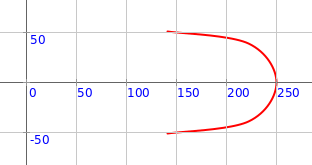Q4a. The code above makes one rounded petal. What is its radius? What is its outer radius? What is its direction (theta)? What is it’s spread (thetaExtent)?

#### Exploration

Play with the inputs to the `roundedPetal` function above to make different kinds of petals.

#### Exercise

Make the following circular pattern: